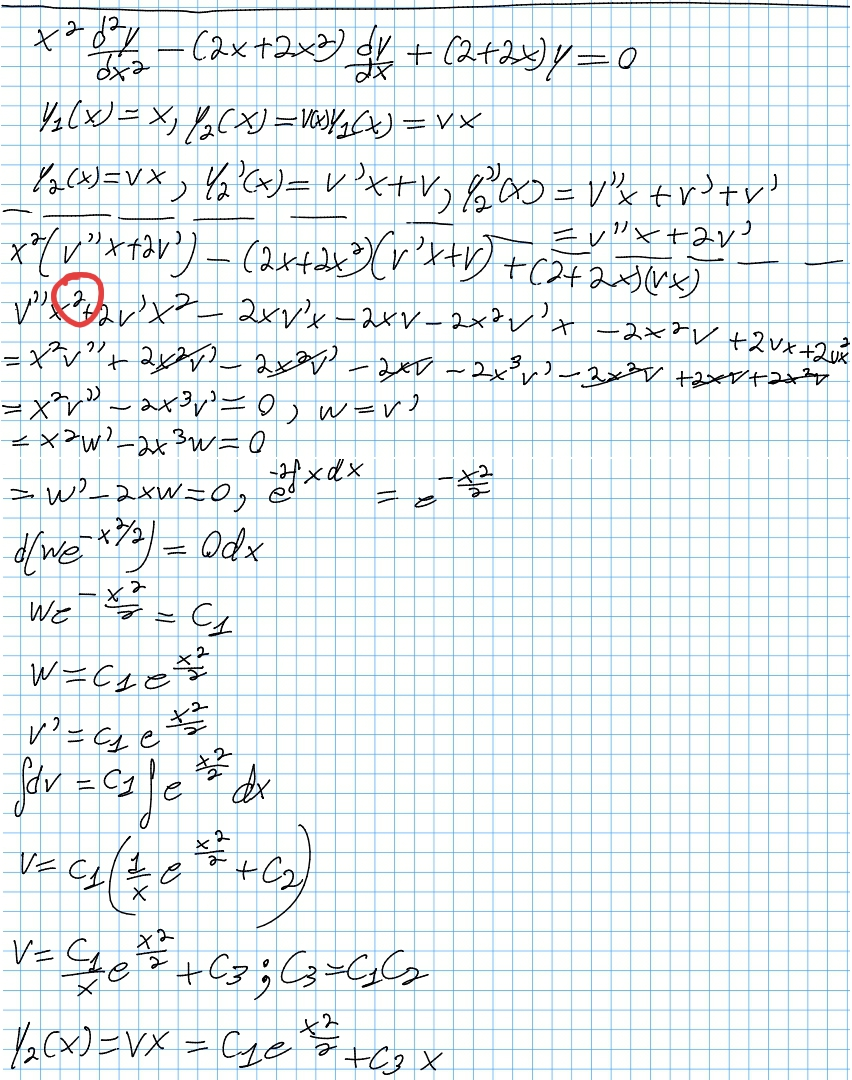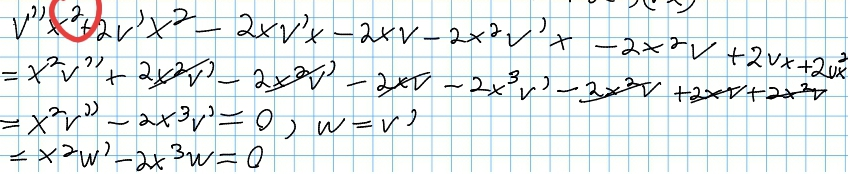# Thread: y_c = ?

1. ## y_c = ?

I am VERY confused with this question. Firstly, whenever the coefficients are variables, I have no idea what to do. I'm thinking divide both sides by x^2 based on something I think I remember but I am really not sure and if that is what I am supposed to do, I'm guessing it would be to make the equation be in some form so I can do something to it but I really need someone to give me the specifics or at least give me the exact names of the methods to use with differential equations such as these which have variable coefficients.

Secondly, what does the subscript c in the final solution stand for and what does what it stands for mean? As for what a fundamental set of solutions is, all I know is that two solutions are said to form a fundamental set of solutions if and only if their Wronskian is nonzero.

I would so greatly appreciate it if someone could outline all the steps (any tedious calculations that have no significant theoretical aspect can be omitted) for me because I am having a lot of trouble.

2. ## Re: y_c = ?

Hint Use the substitution $\displaystyle y=vx$ and denote $\displaystyle z=v'$ . You'll obtain a simple first order equation on $\displaystyle z$ which allows to find $\displaystyle y_c$ .

3. ## Re: y_c = ?Originally Posted by FernandoRevillaHint Use the substitution $\displaystyle y=vx$ and denote $\displaystyle z=v'$ . You'll obtain a simple first order equation on $\displaystyle z$ which allows to find $\displaystyle y_c$ .
A procedure known, at least in the States, as "Reduction of Order". To FernandoRevilla you listen!

4. ## Re: y_c = ?Originally Posted by AckbeetA procedure known, at least in the States, as "Reduction of Order".
However, in Spain it is known as $\displaystyle \textrm{Reducci\'on\;del\;orden}$ . (Literally translated: Reduction of order)5. ## Re: y_c = ?

Ok, I wanted more details than that but what you guys told me did help me know what to look for and I found this:

Pauls Online Notes : Differential Equations - Reduction of Order

and I get it now BUT the question I am asking here, seems to go further than what the link above is saying (as well as what you two are saying) and judging by something I read from my class textbook (which I find very difficult to understand), "fundamental set of solutions" implies Wronskian usage but I don't know what to do specifically and would appreciate further assistance.

For the reduction of order part, I get y_c(x) = y_2(x) = c_1 * e^(x^2 /2) + c_3 * x as the final answer where c_1 and c_3 are constants.

6. ## Re: y_c = ?Originally Posted by s3aFor the reduction of order part, I get y_c(x) = y_2(x) = c_1 * e^(x^2 /2) + c_3 * x as the final answer where c_1 and c_3 are constants.
How so? What did you get for z = w' and z' = w'' ?

Just in case a picture helped with that...... where (key in first spoiler) ...

Spoiler:... is the product rule, straight lines differentiating downwards with respect to x.

Spoiler:

Divide the given equation through by a suitable power of x and you have the bottom row minus twice the middle row...Full size

Did you get that far (probably as a first order equation in z)?

(Remaining pictures I'll keep back, as you probably want to share your version.)

So integrate each section...Full size

... and you can separate variables...

Spoiler:where... (key in first spoiler)

Spoiler:... is the chain rule. Straight continuous lines differentiate downwards (integrate up) with respect to the main variable (in this case x), and the straight dashed line similarly but with respect to the dashed balloon expression (the inner function of the composite which is subject to the chain rule).

Spoiler:Full size

And add the given solution to this one.

__________________________________________________ __________

Don't integrate - balloontegrate!

Balloon Calculus; standard integrals, derivatives and methods

Balloon Calculus Drawing with LaTeX and Asymptote!

7. ## Re: y_c = ?

I appreciate the pictures but I'm more of a variables and numbers kind of guy for most things and I get things like product rule, etc that you illustrated. My entire work is attached.

8. ## Re: y_c = ?

Here's the thing...9. ## Re: y_c = ?

Thank you for finding that but I did it again starting from w' - 2w = 0 since all the other terms cancelled out so my previous mistake just required me to change:

x^2 * w' - 2x^3 * w = 0 to

w' - 2w = 0 (like mentioned above)

and I get it wrong again!

Could you tell me what it is that I am doing wrong this time?
Sorry I'm still relatively new to this.

10. ## Re: y_c = ?

Actually, I found yet another mistake but now I am stuck on the last step of the attached image.

11. ## Re: y_c = ?

Just algebra fatigue. What you had before...... is fine with the power corrected, and does indeed give you

w' - 2w = 0

... and what's wrong with that? What's your solution as y = f(x)?